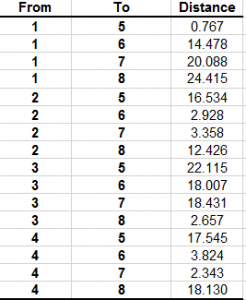In this tutorial, I’ll finish the process of converting a simple Excel Transshipment problem that I wrote to minimize the traveling distance that a set of patrol cars would have to travel between two sets of patrol positions. We want to convert this problem from Excel to R so that we can eventually use R to loop through a series of transfers that are going to occur over a twenty-four hour schedule that covers an entire week.

The R library we’re going to use for the linear program optimization is called lpSolveAPI. First we’ll put down the call to the library:

`library(lpSolveAPI)`

Then we’ll going to set down the number of constraints, the number of decision variables, and the type of error reporting. We have four positions we’re traveling FROM, four positions we’re traveling TO, and we need to model each different combinations of FROM position to TO position so we’re going to have sixteen different decision variables. For the constraints, we’re going to have nine, for reasons I’ll go into below. For the error reporting, we’re going to say, “full,” because this is our first time, so hey, tell me everything. The call to set these parameters is:
``` make.lp(number of constraints, number of decision variables, error reporting) lpmodel<-make.lp(9, 16, "full") ```
Now we’re going to set our objective function, remember we want to minimize the distance these four police vehicles have to travel to the next patrol position. I’m not going to go into how this was calculated because I’m saving that pain for later. For now , the call will consist of basically the same thing as the Excel model, a vector of distances between every combination of points.Which is done like this:

``` set.objfn(lpmodel, c(0.767,14.478,20.088, 24.415, 16.534,2.928,3.358,12.426, 22.115,18.007,18.431,2.657, 17.545,3.824,2.343,18.130)) ```

There’s a few different ways to do the constraints, and there are ones that are much shorter than the ones we’re going to use here, but for instructional purposes we won’t use sparse matrices or any shortcuts. These constraint matrices are to use make sure that each FROM position will give up one car, and one car only and are tied to the above distance matrix. They assume we’re using the same format as the Excel model, where the first four lines are for position FROM one to each different TO, position FROM two to each different TO, and so forth
``` add.constraint(lpmodel, c(1, 1, 1, 1, 0, 0, 0, 0, 0, 0, 0, 0, 0, 0, 0, 0), "=", 1)```

1, 1, 1, 1,
0, 0, 0, 0,
0, 0, 0, 0), “=”, 1)

0, 0, 0, 0,
1, 1, 1, 1,
0, 0, 0, 0), “=”, 1)

0, 0, 0, 0,
0, 0, 0, 0,
1, 1, 1, 1), “=”, 1)

Now we’re going to make sure that the TO positions only will have one car moving to each of them:
``` add.constraint(lpmodel, c(1, 0, 0, 0, 1, 0, 0, 0, 1, 0, 0, 0, 1, 0, 0, 0), "=", 1) add.constraint(lpmodel, c(0, 1, 0, 0, 0, 1, 0, 0, 0, 1, 0, 0, 0, 1, 0, 0), "=", 1) add.constraint(lpmodel, c(0, 0, 1, 0, 0, 0, 1, 0, 0, 0, 1, 0, 0, 0, 1, 0), "=", 1) add.constraint(lpmodel, c(0, 0, 0, 1, 0, 0, 0, 1, 0, 0, 0, 1, 0, 0, 0, 1), "=", 1) Now we're going to add a constraint to make sure, that there the model variables don't go negative: add.constraint(lpmodel, c(1, 1, 1, 1, 1, 1, 1, 1, 1, 1, 1, 1, 1, 1, 1, 1), ">=", 0) And we're going to define the variables as integers, and add something that lpsolve needs to add by defining a columns variable:```

columns<-seq(1, 16)
set.type(lpmodel, columns, type = c(“integer”))

To solve the model, we make this call:
``` solve(lpmodel) ```
To look at the objective:
``` get.objective(lpmodel) ```
Look at which variables were picked for the actual position-to-position transfers:
``` get.variables(lpmodel) ```
We can also print out the lp format of our model for debugging:
``` write.lp(lpmodel, 'model.lp', type='lp') ```
Here’s the .R file for the model. I had to change the file extension to .txt, wordpress is having a conniption about a .R extension:

LP_EXAMPLE_1